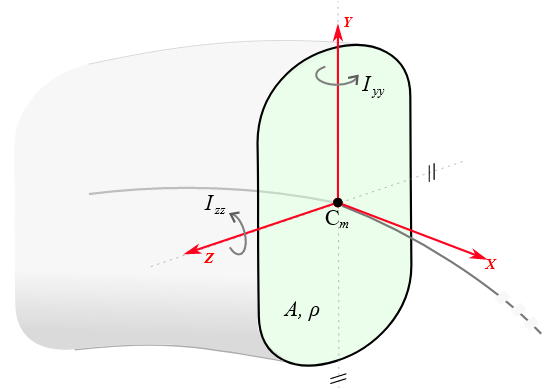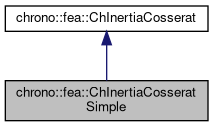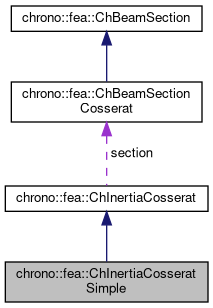chrono::fea::ChInertiaCosseratSimple Class Reference

## Description

Inertia properties of a beam of Cosserat type, defined from an uniform density [kg/m^3], and the following geometric information:

• a section area
• Iyy Izz second moments of area The polar moment of area is automatically inferred via perpendicular axis theorem, Ip=Iyy+Izz. The section is assumed aligned to principal axis of the moment of area tensor, ie. Iyz=0, The section is assumed to be centered in the center of mass, The density is constant.#include <ChBeamSectionCosserat.h>

Inheritance diagram for chrono::fea::ChInertiaCosseratSimple:[legend]
Collaboration diagram for chrono::fea::ChInertiaCosseratSimple:[legend]

## Public Member Functions

ChInertiaCosseratSimple (double density, double Area, double Iyy_area_moment, double Izz_area_moment)

virtual void ComputeInertiaMatrix (ChMatrixNM< double, 6, 6 > &Mi) override
Compute the 6x6 sectional inertia matrix, as in {x_momentum,w_momentum}=[Mi]{xvel,wvel} The matrix is computed in the material reference (i.e. More...

virtual void ComputeInertiaDampingMatrix (ChMatrixNM< double, 6, 6 > &Ri, const ChVector<> &mW) override
Compute the 6x6 sectional inertia damping matrix [Ri] (gyroscopic matrix damping), as in linearization dFi=[Mi]*d{xacc,wacc}+[Ri]*d{xvel,wvel}+[Ki]*d{pos,rot} The matrix is computed in the material reference, i.e. More...

virtual void ComputeInertiaStiffnessMatrix (ChMatrixNM< double, 6, 6 > &Ki, const ChVector<> &mWvel, const ChVector<> &mWacc, const ChVector<> &mXacc) override
Compute the 6x6 sectional inertia stiffness matrix [Ki^], as in linearization dFi=[Mi]*d{xacc,wacc}+[Ri]*d{xvel,wvel}+[Ki]*d{pos,rot} The matrix is computed in the material reference. More...

virtual void ComputeQuadraticTerms (ChVector<> &mF, ChVector<> &mT, const ChVector<> &mW) override
Compute the values of inertial torque depending on quadratic velocity terms, per unit length, that is the gyroscopic torque w x [J]w . More...

virtual double GetMassPerUnitLength () override
Compute mass per unit length, ex.SI units [kg/m] In this case is simply $$\mu = \rho A$$, given area in [m^2] and with $$\rho$$ density in [kg/m^3].

virtual double GetInertiaJxxPerUnitLength ()
Compute the Jxx component of the inertia tensor per unit length, i.e. More...

virtual double GetInertiaJyyPerUnitLength ()
Compute the Jyy component of the inertia tensor per unit length, i.e. More...

virtual double GetInertiaJzzPerUnitLength ()
Compute the Jzz component of the inertia tensor per unit length, i.e. More...

void SetDensity (const double md)
Set the volumetric density, assumed constant in the section. Ex. SI units: [kg/m^3].

double GetDensity () const

void SetArea (const double ma)
Set the area of section for computing mass properties. Ex. SI units: [m^2].

double GetArea () const

void SetIyy (double mi)
Set the Iyy second moment of area of the beam (for bending about y in xz plane), defined as $$I_{yy} = \int_\Omega z^2 d\Omega$$. More...

double GetIyy () const

void SetIzz (double mi)
Set the Izz second moment of area of the beam (for bending about z in xy plane), defined as $$I_{zz} = \int_\Omega y^2 d\Omega$$. More...

double GetIzz () const

virtual void SetAsRectangularSection (double width_y, double width_z, double density)
Shortcut: set Izz, Iyy, Area and density at once, given the y and z widths of the beam assumed with rectangular shape, and volumetric density. More...

virtual void SetAsCircularSection (double diameter, double density)
Shortcut: set Izz, Iyy, Area and density at once, given the diameter the beam assumed with circular shape, and volumetric density. More...Public Member Functions inherited from chrono::fea::ChInertiaCosserat
virtual void ComputeInertialForce (ChVector<> &mFi, ChVector<> &mTi, const ChVector<> &mWvel, const ChVector<> &mWacc, const ChVector<> &mXacc)
Compute the total inertial wrench, ie forces and torques (per unit length). More...

## Additional Inherited MembersPublic Attributes inherited from chrono::fea::ChInertiaCosserat
ChBeamSectionCosseratsection

bool compute_inertia_damping_matrix = true
Flag that turns on/off the computation of the [Ri] 'gyroscopic' inertial damping matrix. More...

bool compute_inertia_stiffness_matrix = true
Flag that turns on/off the computation of the [Ki] inertial stiffness matrix. More...

bool compute_Ri_Ki_by_num_diff = false
Flag for computing the Ri and Ki matrices via numerical differentiation even if an analytical expression is provided. More...

## ◆ ChInertiaCosseratSimple()

 chrono::fea::ChInertiaCosseratSimple::ChInertiaCosseratSimple ( double density, double Area, double Iyy_area_moment, double Izz_area_moment )
inline
Parameters
 density the density fo the material [kg/m^3], assumed constant Area area of the section, [m^2] Iyy_area_moment second moment of area [m^4] about Y Izz_area_moment second moment of area [m^4] about Z

## Member Function Documentation

 void chrono::fea::ChInertiaCosseratSimple::ComputeInertiaDampingMatrix ( ChMatrixNM< double, 6, 6 > & Ri, const ChVector<> & mW )
overridevirtual

Compute the 6x6 sectional inertia damping matrix [Ri] (gyroscopic matrix damping), as in linearization dFi=[Mi]*d{xacc,wacc}+[Ri]*d{xvel,wvel}+[Ki]*d{pos,rot} The matrix is computed in the material reference, i.e.

both linear and rotational coords assumed in the basis of the centerline reference.

Parameters
 Ri 6x6 sectional inertial-damping (gyroscopic damping) matrix values here mW current angular velocity of section, in material frame

Reimplemented from chrono::fea::ChInertiaCosserat.

## ◆ ComputeInertiaMatrix()

 void chrono::fea::ChInertiaCosseratSimple::ComputeInertiaMatrix ( ChMatrixNM< double, 6, 6 > & Mi )
overridevirtual

Compute the 6x6 sectional inertia matrix, as in {x_momentum,w_momentum}=[Mi]{xvel,wvel} The matrix is computed in the material reference (i.e.

it is the sectional mass matrix). In this case it is simply a constant diagonal mass matrix with diagonal {rho*A,rho*A,rho*A, rho*Iyy+Izz, rho*Iyy, rho*Izz}

Parameters
 Mi 6x6 sectional mass matrix values here

Implements chrono::fea::ChInertiaCosserat.

## ◆ ComputeInertiaStiffnessMatrix()

 void chrono::fea::ChInertiaCosseratSimple::ComputeInertiaStiffnessMatrix ( ChMatrixNM< double, 6, 6 > & Ki, const ChVector<> & mWvel, const ChVector<> & mWacc, const ChVector<> & mXacc )
overridevirtual

Compute the 6x6 sectional inertia stiffness matrix [Ki^], as in linearization dFi=[Mi]*d{xacc,wacc}+[Ri]*d{xvel,wvel}+[Ki]*d{pos,rot} The matrix is computed in the material reference.

NOTE the matrix already contains the 'geometric' stiffness, so it transforms to absolute transl/local rot just like [Mi] and [Ri]: [Ki]_al =[R,0;0,I]*[Ki^]*[R',0;0,I'] , with [Ki^]=([Ki]+[0,f~';0,0]) for f=current force part of inertial forces.

Parameters
 Ki 6x6 sectional inertial-stiffness matrix [Ki^] values here mWvel current angular velocity of section, in material frame mWacc current angular acceleration of section, in material frame mXacc current acceleration of section, in material frame (not absolute!)

Reimplemented from chrono::fea::ChInertiaCosserat.

 void chrono::fea::ChInertiaCosseratSimple::ComputeQuadraticTerms ( ChVector<> & mF, ChVector<> & mT, const ChVector<> & mW )
overridevirtual

Compute the values of inertial torque depending on quadratic velocity terms, per unit length, that is the gyroscopic torque w x [J]w .

Quadratic force is null as mass is centered. All terms expressed in the material reference, ie. the reference in the centerline of the section.

Parameters
 mF centrifugal term (if any) returned here mT gyroscopic term returned here mW current angular velocity of section, in material frame

Implements chrono::fea::ChInertiaCosserat.

## ◆ GetInertiaJxxPerUnitLength()

 virtual double chrono::fea::ChInertiaCosseratSimple::GetInertiaJxxPerUnitLength ( )
inlinevirtual

Compute the Jxx component of the inertia tensor per unit length, i.e.

the part associated with rotation about the beam direction. In this case it is $$J_{xx} = \rho I_p$$, where $$I_p = I_{zz} + I_{yy}$$ is the polar moment of area.

## ◆ GetInertiaJyyPerUnitLength()

 virtual double chrono::fea::ChInertiaCosseratSimple::GetInertiaJyyPerUnitLength ( )
inlinevirtual

Compute the Jyy component of the inertia tensor per unit length, i.e.

the part associated with rotation of the section on its Y axis. Defined as: $$J_{yy} = \int_\Omega \rho z^2 d\Omega$$, with $$\rho$$ density in [kg/m^3]. For uniform density it is $$J_{yy} = \rho I_{yy}$$, where $$I_{yy} = \int_\Omega z^2 d\Omega$$ is the second moment of area.

## ◆ GetInertiaJzzPerUnitLength()

 virtual double chrono::fea::ChInertiaCosseratSimple::GetInertiaJzzPerUnitLength ( )
inlinevirtual

Compute the Jzz component of the inertia tensor per unit length, i.e.

the part associated with rotation of the section on its Z axis. Defined as: $$J_{zz} = \int_\Omega \rho y^2 d\Omega$$, with $$\rho$$ density in [kg/m^3]. For uniform density it is $$J_{zz} = \rho I_{zz}$$, where $$I_{zz} = \int_\Omega y^2 d\Omega$$ is the second moment of area.

## ◆ SetAsCircularSection()

 void chrono::fea::ChInertiaCosseratSimple::SetAsCircularSection ( double diameter, double density )
virtual

Shortcut: set Izz, Iyy, Area and density at once, given the diameter the beam assumed with circular shape, and volumetric density.

Assuming centered section.

## ◆ SetAsRectangularSection()

 void chrono::fea::ChInertiaCosseratSimple::SetAsRectangularSection ( double width_y, double width_z, double density )
virtual

Shortcut: set Izz, Iyy, Area and density at once, given the y and z widths of the beam assumed with rectangular shape, and volumetric density.

Assuming centered section.

## ◆ SetIyy()

 void chrono::fea::ChInertiaCosseratSimple::SetIyy ( double mi )
inline

Set the Iyy second moment of area of the beam (for bending about y in xz plane), defined as $$I_{yy} = \int_\Omega z^2 d\Omega$$.

Note: some textbook calls this Iyy as Iy. Note: it can correspond to the same Iyy that you used for the elasticity, ex. in ChElasticityCosseratSimple. Ex. SI units: [m^4]

## ◆ SetIzz()

 void chrono::fea::ChInertiaCosseratSimple::SetIzz ( double mi )
inline

Set the Izz second moment of area of the beam (for bending about z in xy plane), defined as $$I_{zz} = \int_\Omega y^2 d\Omega$$.

Note: some textbook calls this Izz as Iz. Note: it can correspond to the same Izz that you used for the elasticity, ex. in ChElasticityCosseratSimple. Ex. SI units: [m^4]

The documentation for this class was generated from the following files:
• /builds/uwsbel/chrono/src/chrono/fea/ChBeamSectionCosserat.h
• /builds/uwsbel/chrono/src/chrono/fea/ChBeamSectionCosserat.cpp